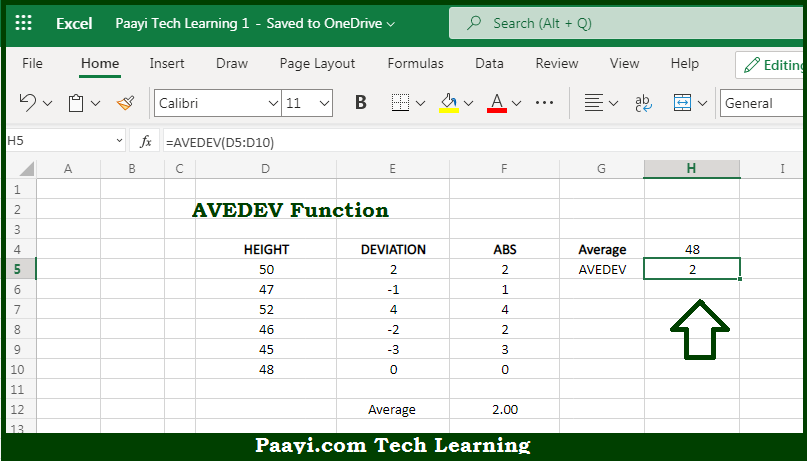# Learn How to Use Microsoft Excel AVEDEV Function

Written by | 0 Comments | 491 Views

In this article, you will learn how to use the Microsoft Excel AVEDEV function and its prime function in Microsoft Excel. You will also get to know the Microsoft Excel AVEDEV function return value and syntax with the help of some examples.

Microsoft Excel AVEDEV Function

The main purpose of the Microsoft Excel AVEDEV function is to get the sum of squared deviations. That implies, with the help of the AVEDEV function you can able to return the average of the absolute value of deviations from the mean for a given set of data. Average deviation can be understood as a measure of variability. So, with the help of the AVEDEV function, you can able to get the sum of the squared deviations.

Return Value of AVEDEV Function

The return value will be the calculated sum.

Syntax of AVEDEV Function

=AVEDEV(number1, [number2], ...)

Where the arguments:

• number1: This is the first value or reference.
• number2: This is the second value or reference (Optional).

## How to Use Microsoft Excel AVEDEV Function?So we know that Microsoft Excel AVEDEV function you can able to get the sum of squared deviations. That implies, with the help of the AVEDEV function you can able to return the average of the absolute value of deviations from the mean for a given set of data. Average deviation can be understood as a measure of variability.

The Microsoft Excel AVEDEV function calculates the average of absolute deviations from the mean in a given set of data. Variance and standard deviation functions work with negative deviations by squaring deviations before their average calculations. AVEDEV handles negative values by working only with absolute values. This average is known as the average absolute deviation. So, with the help of the AVEDEV function, you can able to get the sum of the squared deviations.